# Motion

From two different locations distant 232 km started against car and bus. The car started at 5:20 with average speed 64 km/h. Bus started at 7:30 with average speed 80 km/h.

When they meet?
How many kilometers went the bus?

Result

x (hh:mm) = 8:09s2 =  51.9 km

#### Solution:

$\ \\ \Delta t = (7-5)+\dfrac{1}{60}\cdot (30-20) = 2.17 \ h \ \\ \ \\ s_1 + s_2 = 232 \ \\ v_1(t+\Delta t) + v_2 t = 232 \ \\ \ \\ t = \dfrac{ 232-64 \cdot \Delta t} { 64+80} = 0.65 \ h \ \\ x = 5 + \dfrac{1}{60}\cdot 20 + t + \Delta t = 8.153 \ \\ x = 8:09$
$s_2 = v_2 t = 80 \cdot 0.65 = 51.9 \ \text { km }$

Leave us a comment of this math problem and its solution (i.e. if it is still somewhat unclear...):Be the first to comment!#### Following knowledge from mathematics are needed to solve this word math problem:

Do you have a linear equation or system of equations and looking for its solution? Or do you have quadratic equation? Do you want to convert length units?

## Next similar math problems:

1. TruckIn 7 hours started from town Krnov truck at speed 40 km/h. Passenger car started against it in 8 hours 30 minutes from the city of Jihlava at speed 70 km/h. Distance between this two cities is 225 km. At what time and at what distance from Krnov this two.
2. Train from PragueFirst train from Prague started at 8:00 hour at 40 kilometers per hour. Train from Ostrava started at 9:20 at 80 km per hour. In how many hours and how far from cities with trains meet if the distance of cities is 400 km.
3. Motion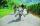Cyclist started at 9:00 from point S to point T. After 10 minutes, followed him at the same speed the second cyclist. Walker, which went from T to S, started at 9:35. After 71 minutes he met the first cyclist and after next 8 minutes the second cyclist. Di
4. Motion2Cyclist started out of town at 19 km/h. After 0.7 hours car started behind him in the same direction and caught up with him for 23 minutes. How fast and how long went car from the city to caught cyclist?
5. Tourists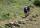From the cottage started first group of tourists at 10:00 AM at speed 4 km/h. The second started after them 47 minutes later at speed 6 km/h. How long and how many kilometers from the cottage will catch the first group?
6. TrainsFrom station 130 km away started passenger train and after 2.2 hours after the express train, which travels 37 km an hour more. Express train finish journey 7 minutes early. Calculate the average speed of this two trains.
7. The cyclist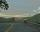The cyclist went from village to town. First half of journey went at 20 km/h. The second half of the journey, which mostly fell, went at 39 km/h. All journey took 88 minutes. Calculate the distance from the village to the town.
8. Scouts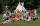The boys from scout group traveled 5 days distance 115 km. Every day walked 1.5 km less than the previous day. How many kilometers scouts walked in the first day?
9. The carThe car has traveled the distance between A and B for four hour. If we increased the average by 17 km/h the car travel this distance an hour earlier. Determine the initial speed of the car and the distance between A and B.
10. Train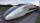A passenger train traveled for 2 hours 74 km. 3.1 hours after its departure started fast train and caught it on 186 km. How many km/h is different its average speeds?
11. Cyclists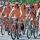Cyclist who rides at an average speed 16 km/h travels trip distance 10 min before the cyclist who rides at an average speed 11 km/h. What is the length of this cyclist trip(distance in km)?
12. Truck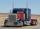Truck went for 4 hours and 30 minutes. If truck increase speed by 11 km/h, went same distance in 2 hours. Calculate this distance.
13. Bike rideMarek rode a bike ride. In an hour, John followed him on the same route by car, at an average speed of 72 km/h, and in 20 minutes he drove him. Will he determine the length of the way that Marek took before John caught up with him, and at what speed did Ma
14. The escalator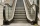I run up the escalator at a constant speed in the direction of the stairs and write down the number of steps A we climbed. Then we turn around and run it at the same constant speed in the opposite direction and write down the number of steps B that I climb
15. MotionIf you go at speed 3.7 km/h, you come to the station 42 minutes after leaving the train. If you go by bike to the station at speed 27 km/h, you come to the station 56 minutes before its departure. How far is the train station?
16. Pedestrian up-down hill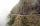Pedestrian goes for a walk first at plane at 4 km/h, then uphill 3 km/h. Then it is in the middle of the route, turns back and goes downhill at speed 6 km/h. Total walk was 6 hours. How many kilometers went pedestrian?
17. Ships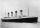At 8:40 the ship set sail at 12 km/h. At 19:10 followed by at 29 km/h sail boat. When sail boat catches up the ship? How many minutes will catch up took?